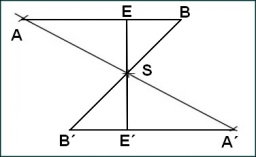# In triangle 2

In triangle XYZ, if measure angle X=40° and measure angle Y=75°, which is the longest side of the triangle and why?

Result

m = (Correct answer is: y)### Step-by-step explanation:Did you find an error or inaccuracy? Feel free to write us. Thank you!

Showing 1 comment:
Dr Math
Hint: The longest side in a triangle is opposite the largest angle, and the shortest side is opposite the smallest angle.

Tips for related online calculators